# Electric potential concepts (1 Viewer)

### Users Who Are Viewing This Thread (Users: 0, Guests: 1)

#### musicfairy

I need some help on those multiple choice questions that I'm using to learn the concepts. Some of them I know how to do and needs someone to check my work. Other ones I need hints so I know how to start.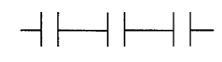1. Three ½ μF capacitors are connected in series as shown in the diagram above. The capacitance of the combination is
(A) 3/2 μF
(B) 1 μF
(C) 2/3 μF
(D) 1/2 μF
(E) 1/6 μF

I used this equation: 1/Ceq = 1/C1 + 1/C2 + 1/C3 and got E as the answer.

2. In a certain region, the electric field along the x-axis is given by

E = ax + b, where a = 40 V/m2
and b = 4 V/m.

The potential difference between the origin and x = 0.5 m is
(A) -36 V
(B) -7 V
(C) -3 V
(D) 10 V
(E) 16 V

I tried to plug in 0 and 0.5 for x and subtract them but that didn't work out.

3. A 20 μF parallel-plate capacitor is fully charged to 30 V. The energy stored in the capacitor is most nearly
(A) 9x103 J
(B) 9xl0-3 J
(C) 6x10-4 J
(D) 2x10-4 J
(E) 2x10-7 J

Used the equation U = (1/2)CV2 and got B as the answer.

4. A potential difference V is maintained between two large, parallel conducting plates. An electron starts from rest on the surface of one plate and accelerates toward the other. Its speed as it reaches the second plate is proportional to
(A) 1/V
(B) 1 / √V
(C) √V
(D) V
(E) V2

qV = (1/2)mv2

When I rearrange it to isolate v it's proportional to √V so it's C.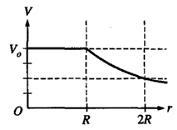5. A solid metallic sphere of radius R has charge Q uniformly distributed on its outer surface. A graph of electric potential V as a function of position r is shown above. Which of the following graphs best represents the magnitude of the electric field E as a function of position r for this sphere?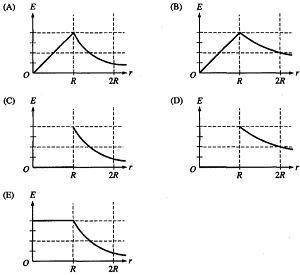Electric field in a conductor is 0 and is proportional to 1/r2, so C is the answer.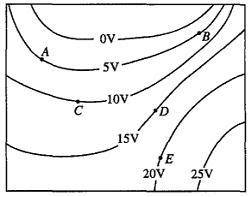This diagram is used for the next 3.

6. Which vector below best describes the direction of the electric field at point A?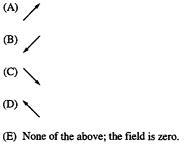Electric field directs toward lower potential, so it's D???

7. At which point does the electric field have the greatest magnitude?

(A) A
(B) B
(C) C
(D) D
(E) E

Not sure. How do I start?

8. How much net work must be done by an external force to move a -1 μC point charge from rest at point C to rest at point E?

(A) -20 μJ
(B) -10 μJ
(C) 10 μJ
(D) 20 μJ
(E) 30 μJ

W = qV
= -1(20 - 10)
= -10 μJ

It's B.

Can someone(s) also please explain to me when is work done by field positive or negative? Thanks.

#### Delphi51

Homework Helper
E = dV/dx. So in #2 to get the V you must integrate Edx.
In #6, wouldn't it be A that points toward the lower V - perpendicular to the V line?
In #7, you want the place where the lines of constant potential are closest together so the dV/dx is highest.

#### musicfairy

So you mean that in #7 B is the correct answer since the potential lines are the closest?

Homework Helper
Yes.

How do I do #2?

#### Delphi51

Homework Helper
in #2 to get the V you must integrate Edx.
Use the expression you have for E and perform the integration.
If you have trouble, show your work here so we can see what is going on.

#### musicfairy

Ok, I see.

V = −∫E∙dr
= −ax2/2 + bx
= −20x2 − 4x

When x = 0.5
V = −7, so B is the answer.

Is this correct?

#### Delphi51

Homework Helper
Yes, that's what I get.

#### musicfairy

Thank you for your help.

### The Physics Forums Way

We Value Quality
• Topics based on mainstream science
• Proper English grammar and spelling
We Value Civility
• Positive and compassionate attitudes
• Patience while debating
We Value Productivity
• Disciplined to remain on-topic
• Recognition of own weaknesses
• Solo and co-op problem solving